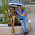## martedì 17 aprile 2007

### From Similarity To Distance matrix

``` # This function returns an object of class "dist" sim2dist <- function(mx) as.dist(sqrt(outer(diag(mx), diag(mx), "+") - 2*mx)) # from similarity to distance matrix d.mx = as.matrix(d.mx) d.mx = sim2dist(d.mx) # The distance matrix can be used to visualize # hierarchical clustering results as dendrograms hc = hclust(d.mx) plot(hc)```

See Multivariate Analysis (Probability and Mathematical Statistics)for the statistical theory.

#### 4 commenti:

1.Thank you a lot!

(I suppose s.mx should be d.mx)

Dotoma

2.You're right! Fixed! Thank you!

3.which page/section is the theory? Thank you!

4.Dear Jianhua, this post was written long time ago (2007) and unfortunately I don't have access to that book anymore...
The RFLPtools package seems to present a similar function, take a loot at http://rgm3.lab.nig.ac.jp/RGM/r_function?p=RFLPtools&f=sim2dist as a starting point. HIH!